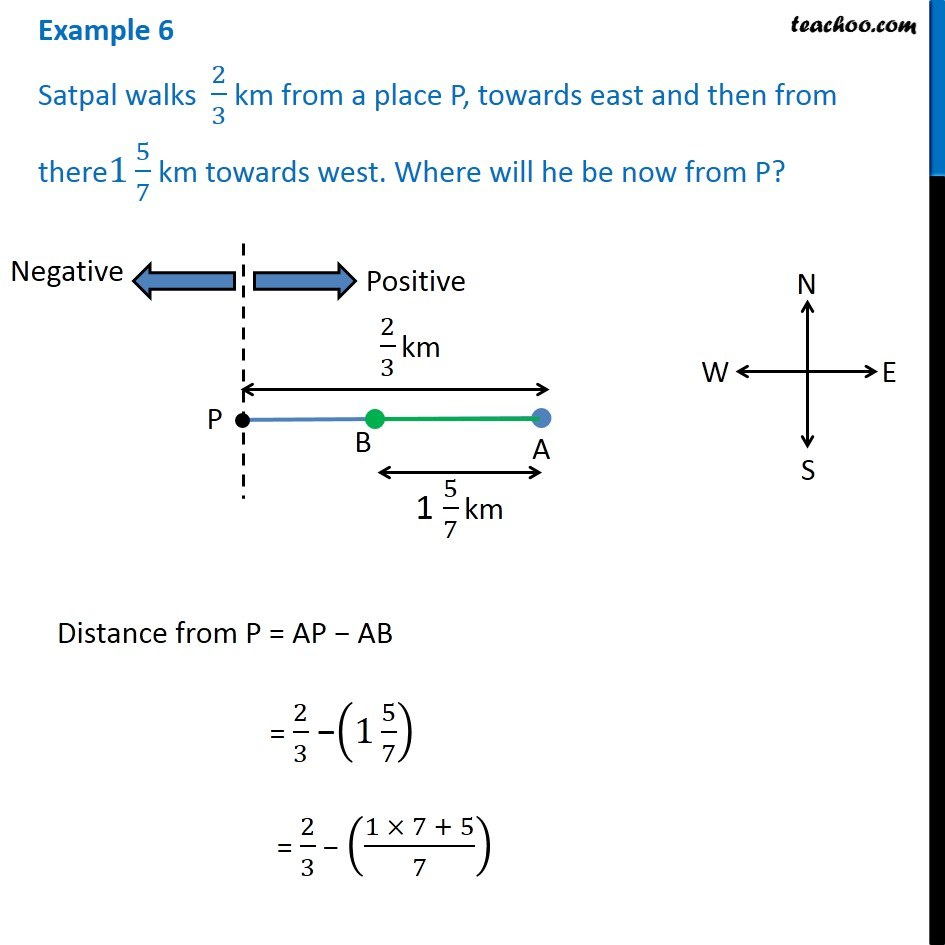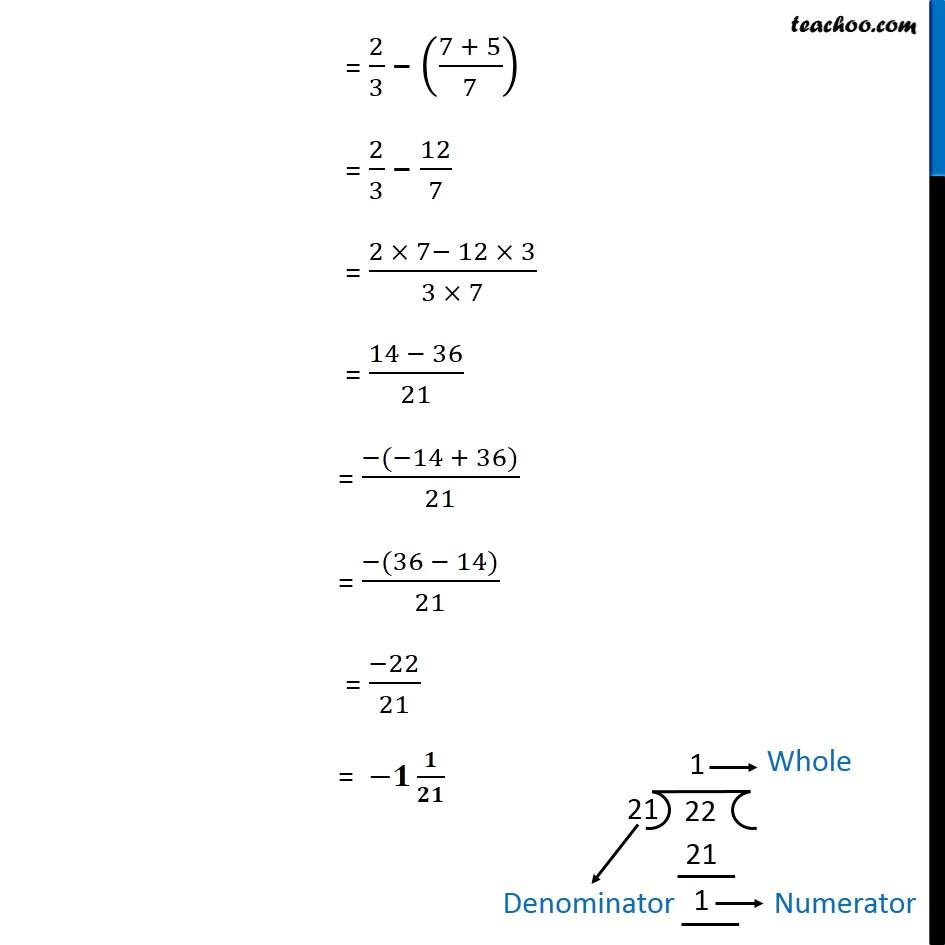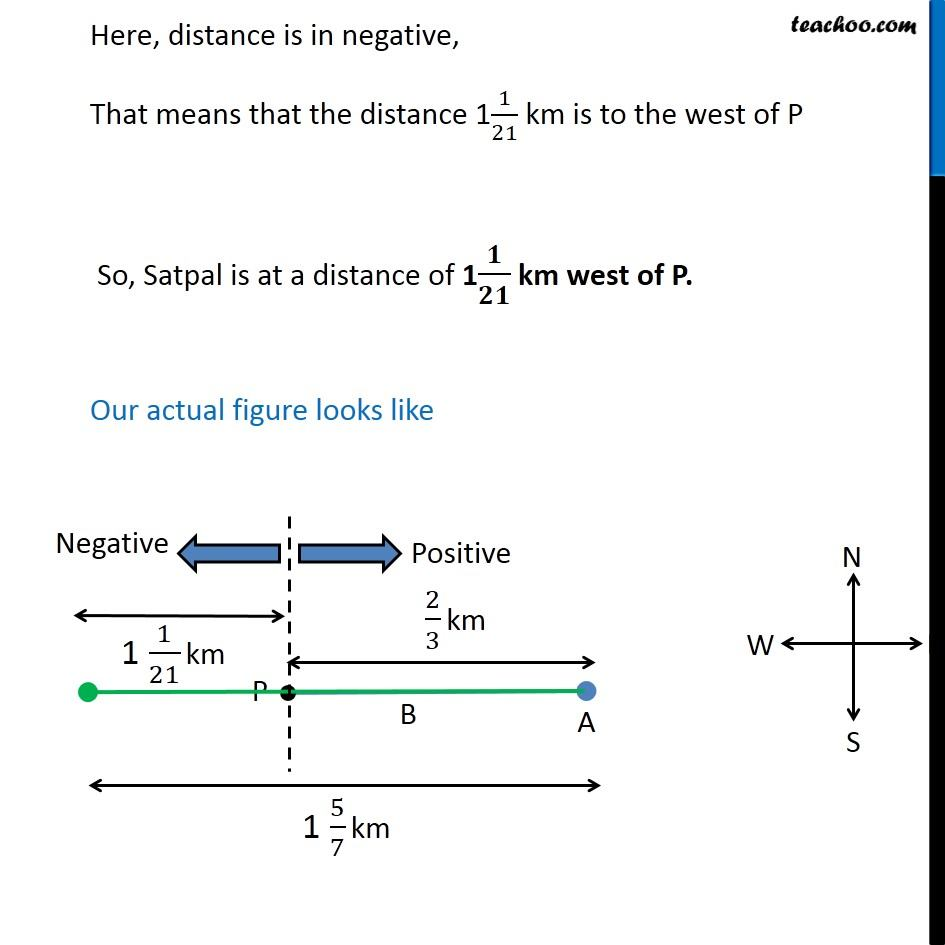1. Chapter 9 Class 7 Rational Numbers
2. Concept wise
3. Addition & Subtraction of rational numbers

Transcript

Example 6 Satpal walks 2/3 km from a place P, towards east and then from there 1 5/7 km towards west. Where will he be now from P? Distance from P = AP AB = 2/3 (1 5/7) = 2/3 ((1 7 + 5)/7) = 2/3 ((7 + 5)/7) = 2/3 12/7 = (2 7 12 3)/(3 7) = (14 36)/21 = ( ( 14 + 36))/21 = ( (36 14))/21 = ( 22)/21 = / Here, distance is in negative, That means that the distance 11/21 km is to the west of P So, Satpal is at a distance of 1 / km west of P. Our actual figure looks like

Addition & Subtraction of rational numbers